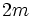# Split orthogonal group

## Definition

This group is the orthogonal group for a symmetric bilinear form where the symmetric bilinear form gives a hyperbolic space.

Let$m$ be a natural number and$k$ be any field. The split orthogonal group of degree$2m$ over$k$ can be defined as the group, under matrix multiplication:$\{ A \in GL(2m,k) \mid A \begin{pmatrix} 0 & I \\ I & 0 \\\end{pmatrix}A^T = \begin{pmatrix} 0 & I \\ I & 0 \\\end{pmatrix} \}$.

Here, the$0$ and$I$ are$n \times n$ block matrices.

When the characteristic of$k$ is not equal to two, this is isomorphic to the group (in fact, they are conjugate in$GL(2m,k)$):$\{ A \in GL(2m,k) \mid A \begin{pmatrix} I & 0 \\ 0 & -I \\\end{pmatrix}A^T = \begin{pmatrix} I & 0 \\ 0 & -I \\\end{pmatrix} \}$.

For a finite field, the split orthogonal group is also sometimes known as the orthogonal group of$+$ type or the$+1$ type.

## Particular cases

### Finite fields

Size of field$m$$2m$ Common name for the split orthogonal group Order of the group
3 1 2 Klein four-group 4
5 1 2 dihedral group:D8 8
7 1 2 direct product of S3 and Z2 12
9 1 2 dihedral group:D16 16